## Sign of the magnitude differenceStarted by 4 years ago20 replieslatest reply 4 years ago219 views

Hi ,

Consider two complex numbers  Z1 and Z2 and the following definition of  magnitude difference

|z1| - |z2| --------- (1)   First compute mag and then difference

|z1- z2|  ----------- (2)  First difference (I1-I2 , Q1-Q2) and then mag.

Its obvious that mag difference  by definition of 2 will never go negative whereas mag difference defined by (1) can go negative. Also both are not equal.

My question is if have phase and magnitude of (2) is there way to calculte the sign of mag difference defned by 1.

Regards

Sudarshan

[ - ]I do not understand: the phase  of (2) is always 0.  If the given is strictly |z1-z2| , then forget it you will never be able to find out which of z1 or z2 has the highest modulus (as a counter, simplest,  example take z1 and z2 in phase and then exchange index 1 and 2). If you mean knowing the amplitude |z1-z2| of (z1-z2) AND its phase Arg(z1-z2) .. then yes, I think 'yes you can'.

[ - ]Hi ,

I am not sure why you would say phase of (2) is always zero it can have finite phase depending on the numbers.

"If you mean knowing the amplitude |z1-z2| of (z1-z2) AND its phase Arg(z1-z2) .. then yes, I think 'yes you can"  Knowing these two how is it possible to calculte |z1| - |z2| . Would be really helpful if you can elaborate.

[ - ]Well to be sure let me recall your definition: "|z1- z2|  (2)  First difference (I1-I2 , Q1-Q2) and then mag. "  The "then mag" is important, if the difference of z1-z2 is a complex (Re,Im) or (mag,phase) when you take the magnitude |z1-z2| .. well you obtain a real, and even more a positive real, so its phase is always zero.

[ - ]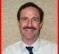Equation 1 calculates the magnitude difference ignoring the phases of z1 and z2.  I would consider that difference to be incorrect because of this.

Equation 2 calculates the magnitude of the difference between the actual complex signals.  This is the correct way to do it.

[ - ]Hi Greg,

Agree with your assertion that eq 2 is correct way . But i am looking for a way to get the sign of |z1| - |z2| given mag and phase of z1-z2.

[ - ]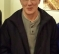Sudarshan-

So what are really saying ?  You cannot simply calculate |z1| -|z2|, for whatever reason ?  I.e. you no longer have z1 and z2 separately, but you do have a number for z1 - z2 ?

Like say you inherited some code / firmware and it discards actual z1 and z2 values and all you got left is the result from equation 2 ?

Is that the actual problem ?

-Jeff

[ - ]Hi Jeff,

Exactly !! I dont have original z1 and z2 and have only magnitude and phase of z1-z2 . Is there anyway to retrieve the sign of |z1| - |z2| or essentially know whose magnitude was higher.

Regards

Sudarshan

[ - ]Sudarshan-

Hmm seems like they stuck you on a legacy system.  Well, that is engineering, it happens to everyone :)

To think about this, consider just real numbers.  If my system subtracts two numbers and burps up a "5" and then hands over that 5 to you without any other info, it's gonna be very hard for you to guess what numbers I had.  But, if you know anything else about my system -- for example when the number I give you is small, the system tends to be in some particular mode, when the number I give you is large, some other mode, if the rate at which I give you numbers varies and that is meaningful, if the delta (rate of change) means something, etc -- then maybe you can start to make educated guesses at my original 2 numbers.   At least to the point of guessing which is bigger, which seems to be your goal.

-Jeff

[ - ]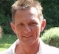Solve it graphically:

draw a vector z1 on a paper: let's say quadrant 1, starting from (0|0).
then draw a vector z2 starting from the endpoint of z1, into any other direction and with arbitrary length.
Now connect (0|0) to the endpoint of the z2-line and you have a vector (z1-z2).

So, if I got you right, all you have is this last vector.
And your statement is, that you want to find out by which two other vectors this last one must have been constructed.
Let me tell you that there is an infinite number of possibilities for two vectors which would result in this (z1-z2) vectors, therefore you can certainly not tell anything about a single one of them, and nothing about their angles or lengths.

[ - ]Hi sudarshan. I am confused by the words you used in your post. Three times you used the phrase "mag difference." If I replace your word "mag" with the word "number", your phrase becomes "number difference", which does not make sense to me.

Would it be correct to change the wording of your question in your last sentence to, "If I have the magnitude and phase of quantity z1-z2, is there way determine which is larger, |z1| or |z2|?"

[ - ]Hi. To "follow-up" on my earlier June 10th post where I suggested rephrasing sudarshan's question to:

"If I have the magnitude and phase of quantity z1-z2, is there way determine which is larger, |z1| or |z2|?"

If my rephrased question is a valid question, then I believe the answer to my rephrased question is "No."

[ - ]Hi Rick ,

Thanks for correcting the phrase.  Your rephrased question is correct.

Now is the answer No in all circumstances or is it possible put some constraint and get an approximation. In short is it worthwhile to pursue this ?

Regards

Sudarshan

[ - ]Hi sudarshan_onkar. Your original post's question was interesting to me. Yesterday I didn't see any straightforward algebra solution to your problem, so I started to plot various vectors on a complex plane.

As far as I can tell, I agree with bholzmayer's June 11th post. That is, given only that you know the magnitude and phase of quantity z1-z2, there is NO way to determine which is larger, |z1| or |z2|. Here's my justification for my last sentence; the following diagram is for an "example" z1 that I will call 'z1a' (the bold blue arrow) having a magnitude of 10 and an angle of 165 degrees.The following diagram is for another "example" z1 that I will call 'z1b' having a magnitude of 10 and an angle of 65 degrees.If I superimpose the above two diagrams into the following diagram you can see that given the complex value of point 'A' we don't know which of the following conditions is true:

Is |z2| > |z1a|?
or
Is |z2| < |z1b|.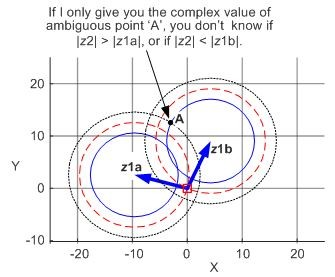That uncertainty is why I say, "Given only that you know the magnitude and phase of quantity z1-z2, there is NO way to determine which is larger, |z1| or |z2|. [I hope my diagrams make sense!]

sudarshan, you wrote "Now is the answer No in all circumstances or is it possible put some constraint and get an approximation." I believe the answer is NO in all circumstances. As for what constraints could be placed on z1 or z2 so that the answer is "Yes", that is a pretty tough question for which I have no answer.

[ - ]Hi Sudarshan, may not be fully inline with your problem statement. however, here a formula which may help at least in another similar situation.

take the part of the problem statement as you defined : that (1) and (2) are given along with the phase differences. then, it is possible to find the absolute values of z1 and z2 ...using basic trig identities.eqn(2) factor '2' is missing. pl read it as 2.r1.r2.cos(dt) instead of r1.r2.cos(dt)

[ - ]Hi chalil. Can you give us more details on how you arrived at your Equation 2 (your equation for y squared)? Thanks.

[ - ]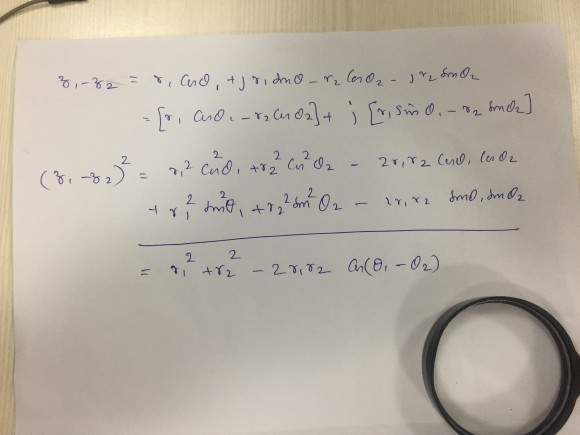there was a typo(factor 2 was missing) in one of the steps my original image.

[ - ]Hi chalil.  Regarding your June 13th Reply, I think there's a missing 2 in your 4th line down, but your bottom line is correct. In any case I think the derivation in your June 12th image is neat. Good Job!!

[I hope sudarshan_onkar has looked at the recent Replies on this thread.]

[ - ]Thank you Rick.

[ - ]Hi Rick,

Thanks for your responses and for a good discussion .  I was away for a month so only  looking now.

[ - ]